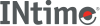﻿ log1pINtime SDK Help
log1p

Computes loge(1.0 + `x`x).

```#include <math.h>

double log1p (double x);
```

#### Return Values

Upon successful completion, this function shall return the natural logarithm of 1.0 + `x`.

If `x` is -1, a pole error shall occur and log1p() shall return -HUGE_VAL.

For finite values of `x` that are less than -1, or if `x` is -Inf, a domain error shall occur, and a NaN shall be returned.

If `x` is NaN, a NaN shall be returned.

If `x` is +/-0, or +Inf, `x` shall be returned.

If `x` is subnormal, a range error may occur and `x` should be returned.

#### Requirements

Versions Defined in Include Link to
INtime 3.0 intime/rt/include/math.h math.h clib.lib